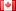# Revision history [back]

### Finding posets of a certain form 2

I try to obtain the connected posets with n elements having a top and bottom such that in the Hasse quiver, in every point there start at most two arrows and at every point there end at most two arrows (thus for example all distributive lattices are of this form). Is there a quick command to obtain this as a list? What condition does one has to add in posets = [ p for p in Posets(n) if p.is_connected() and p.has_top() and p.has_bottom()] ?

### Finding posets of a certain form 2

I try to obtain the connected posets with n elements having a top and bottom such that in the Hasse quiver, in every point there start at most two arrows and at every point there end at most two arrows (thus for example all distributive lattices are of this form). Is there a quick command to obtain this as a list? What condition does one has to add in posets = [ p for p in Posets(n) if p.is_connected() and p.has_top() and p.has_bottom()] ?? Actually a quick method would be cool which works up to n=10 or 11. (filtering things out of the set of all posets, seems to be very slow in SAGE)3 Nonefidbc2298 ●4 ●24 ●55

### Finding posets of a certain form 2

I try to obtain the connected posets with n elements having a top and bottom such that in the Hasse quiver, in every point there start at most two arrows and at every point there end at most two arrows (thus for example all distributive lattices are of this form). Is there a quick command to obtain this as a list? What condition does one has to add in in

posets    = [ p for p in Posets(n) if p.is_connected() and p.has_top() and p.has_bottom()] ? p.has_bottom()]


Actually a quick method would be cool which works up to n=10 or 11. (filtering things out of the set of all posets, seems to be very slow in SAGE)4 Nonefidbc2298 ●4 ●24 ●55

### Finding posets of a certain form 2

I try to obtain the connected posets with n elements having a top and bottom such that in the Hasse quiver, in every point there start at most two arrows and at every point there end at most two arrows (thus for example all distributive lattices are of this form). Is there a quick command to obtain this as a list? What condition does one has to add in

posets  = [ p for p in Posets(n) if p.is_connected() and p.has_top() and p.has_bottom()]


Actually a quick method would be cool which works up to n=10 or 11. (filtering things out of the set of all posets, seems to be very slow in SAGE)5 retaggedFrédéricC4383 ●3 ●37 ●93

### Finding posets of a certain form 2

I try to obtain the connected posets with n elements having a top and bottom such that in the Hasse quiver, in every point there start at most two arrows and at every point there end at most two arrows (thus for example all distributive lattices are of this form). Is there a quick command to obtain this as a list? What condition does one has to add in

posets = [ p for p in Posets(n) if p.is_connected() and p.has_top() and p.has_bottom()]


Actually a quick method would be cool which works up to n=10 or 11. (filtering things out of the set of all posets, seems to be very slow in SAGE)6 retaggedFrédéricC4383 ●3 ●37 ●93

### Finding posets of a certain form 2

I try to obtain the connected posets with n elements having a top and bottom such that in the Hasse quiver, in every point there start at most two arrows and at every point there end at most two arrows (thus for example all distributive lattices are of this form). Is there a quick command to obtain this as a list? What condition does one has to add in

posets = [ p for p in Posets(n) if p.is_connected() and p.has_top() and p.has_bottom()]


Actually a quick method would be cool which works up to n=10 or 11. (filtering things out of the set of all posets, seems to be very slow in SAGE)7 retaggedFrédéricC4383 ●3 ●37 ●93

### Finding posets of a certain form 2

I try to obtain the connected posets with n elements having a top and bottom such that in the Hasse quiver, in every point there start at most two arrows and at every point there end at most two arrows (thus for example all distributive lattices are of this form). Is there a quick command to obtain this as a list? What condition does one has to add in

posets = [ p for p in Posets(n) if p.is_connected() and p.has_top() and p.has_bottom()]


Actually a quick method would be cool which works up to n=10 or 11. (filtering things out of the set of all posets, seems to be very slow in SAGE)